### 2.3.1.1 Backward value iteration

As for the search methods, there are both forward and backward versions of the approach. The backward case will be covered first. Even though it may appear superficially to be easier to progress from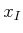, it turns out that progressing backward from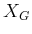is notationally simpler. The forward case will then be covered once some additional notation is introduced.

The key to deriving long optimal plans from shorter ones lies in the construction of optimal cost-to-go functions over. Forfromto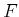, let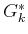denote the cost that accumulates from stagetounder the execution of the optimal plan: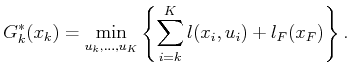(2.5)

Inside of the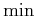of (2.5) are the last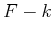terms of the cost functional, (2.4). The optimal cost-to-go for the boundary condition of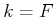reduces to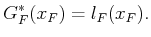(2.6)

This makes intuitive sense: Since there are no stages in which an action can be applied, the final stage cost is immediately received.

Now consider an algorithm that makes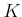passes over, each time computingfrom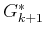, asranges fromdown to. In the first iteration,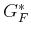is copied from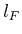without significant effort. In the second iteration,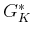is computed for each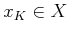as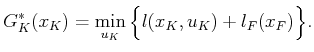(2.7)

Since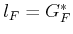and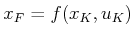, substitutions can be made into (2.7) to obtain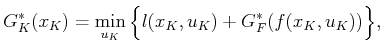(2.8)

which is straightforward to compute for each. This computes the costs of all optimal one-step plans from stageto stage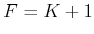.

It will be shown next thatcan be computed similarly onceis given. Carefully study (2.5) and note that it can be written as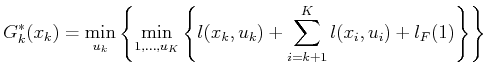(2.9)

by pulling the first term out of the sum and by separating the minimization over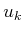from the rest, which range from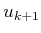to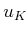. The seconddoes not affect the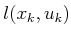term; thus,can be pulled outside to obtain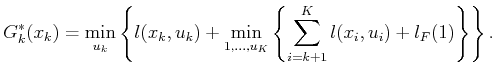(2.10)

The inneris exactly the definition of the optimal cost-to-go function. Upon substitution, this yields the recurrence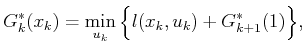(2.11)

in which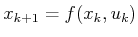. Now that the right side of (2.11) depends only on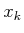,, and, the computation ofeasily proceeds in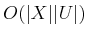time. This computation is called a value iteration. Note that in each value iteration, some states receive an infinite value only because they are not reachable; a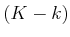-step plan fromtodoes not exist. This means that there are no actions,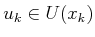, that bringto a state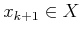from which a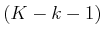-step plan exists that terminates in.

Summarizing, the value iterations proceed as follows: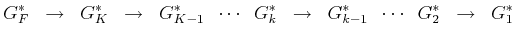(2.12)

until finally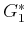is determined after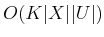time. The resultingmay be applied to yield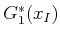, the optimal cost to go to the goal from. It also conveniently gives the optimal cost-to-go from any other initial state. This cost is infinity for states from whichcannot be reached instages.

It seems convenient that the cost of the optimal plan can be computed so easily, but how is the actual plan extracted? One possibility is to store the action that satisfied thein (2.11) from every state, and at every stage. Unfortunately, this requires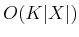storage, but it can be reduced to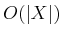using the tricks to come in Section 2.3.2 for the more general case of variable-length plans.

Example 2..3 (A Five-State Optimal Planning Problem)   Figure 2.8 shows a graph representation of a planning problem in which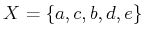. Suppose that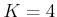,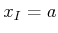, and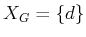. There will hence be four value iterations, which construct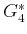,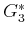,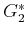, and, once the final-stage cost-to-go,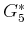, is given.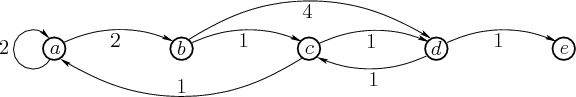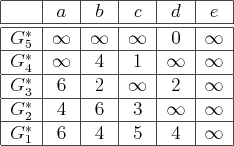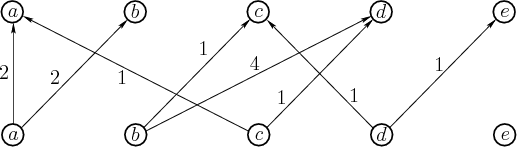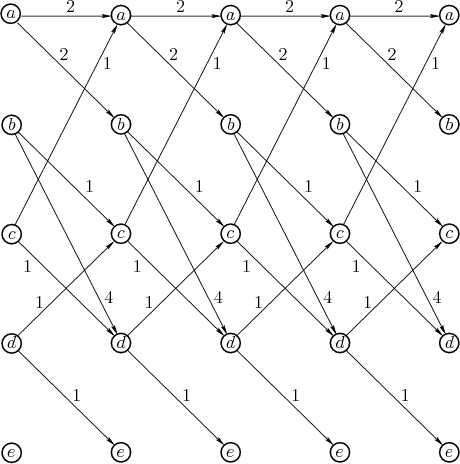The cost-to-go functions are shown in Figure 2.9. Figures 2.10 and 2.11 illustrate the computations. For computing, only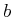and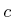receive finite values because only they can reach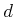in one stage. For computing, only the values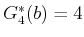and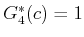are important. Only paths that reachorcan possibly lead toin stage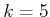. Note that the minimization in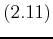always chooses the action that produces the lowest total cost when arriving at a vertex in the next stage.Steven M LaValle 2020-08-14